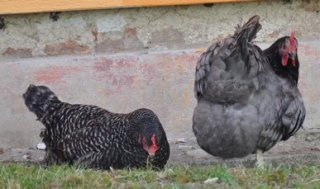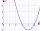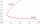# Chickens and rabbits

In the yard were chickens and rabbits. Together they had 51 heads and 122 legs. How many chickens and how many rabbits was in the yard?

Result

chickens:  41
rabbits:  10

#### Solution:

$a+b = 51 \ \\ 2a+4b=122 \ \\ a=41 \ \\ b=10$

a+b = 51
2*a+4*b=122

a+b = 51
2a+4b = 122

a = 41
b = 10

Calculated by our linear equations calculator.Our examples were largely sent or created by pupils and students themselves. Therefore, we would be pleased if you could send us any errors you found, spelling mistakes, or rephasing the example. Thank you!

Leave us a comment of this math problem and its solution (i.e. if it is still somewhat unclear...):Math student
We know that chicken has 2 legs and rabbit has 4. Assume that all heads are of chicken = 44*2 = 88. Now the difference between the total no. Of legs = 124-88 = 36. 36/2 =18 legs of Rabbits and 44-18 = 26 for chicken

1 year ago  15 LikesMath student
Imagine all rabbits and chickens are well trained. We blow the whistle, all chickens and rabbits would retract one of their legs. 124 - 44 = 80. Then we blow the whistle again, they retract another leg. 80-44= 36. these remaining 36 legs belong to the rabbits which are standing with two legs (the other two are retracted). thus 36/2 = 18 rabbits. and the chickens are 44-18 = 26.

2 years ago  5 LikesMath student
viju has 40 chickens and rabbits.  If in all there are 90 legs.  How many rabbits are there with viju??

3 years ago  11 LikesManikanta
35 chicken 5 rabbit

2 years ago  6 LikesMath student
Imagine all rabbits and chickens are well trained. We blow the whistle, all chickens and rabbits would retract one of their legs. 124 - 44 = 80. Then we blow the whistle again, they retract another leg. 80-44= 36. these remaining 36 legs belong to the rabbits which are standing with two legs (the other two are retracted). thus 36/2 = 18 rabbits. and the chickens are 44-18 = 26.

2 years ago  5 LikesMath student
We know that chicken has 2 legs and rabbit has 4. Assume that all heads are of chicken = 44*2 = 88. Now the difference between the total no. Of legs = 124-88 = 36. 36/2 =18 legs of Rabbits and 44-18 = 26 for chicken

1 year ago  15 LikesMath student
35 chiken and 5 rabit

1 year ago  3 LikesMath student
Nonsense question is something answering something elseMath student
40 chickens means 80 legs rest 10 legs .rabbit has 4 legs or 2 legs?10 legs will be shared among rabbit.Tips to related online calculators
Do you have a linear equation or system of equations and looking for its solution? Or do you have quadratic equation?

## Next similar math problems:

1. Collection of stampsJano, Rado, and Fero have created a collection of stamps in a ratio of 5: 6: 9. Two of them had 429 stamps together. How many stamps did their shared collection have?
2. DozenWhat is the product of 26 and 5? Write the answer in Arabic numeral. Add up the digits. How many of this is in a dozen? Divide #114 by this
3. Evaluate 5Evaluate expression x2−7x+12x−4 when x=−1
4. Two integersTwo integers, a and b, have a product of 36. What is the least possible sum of a and b?
5. Evaluate - order of opsEvaluate the expression: 32+2[5×(24-6)]-48÷24 Pay attention to the order of operation including integersIn about 12 hours in North Dakota the temperature rose from -33 degrees farenheit to 50 degrees farenheit. By how much did the temperature change?
7. The differenceThe difference of two numbers is 1375. If their exact quotient is 12. Find the two numbers
8. Expression plus minusEvaluate expression: (-1)2 . 12 – 6 : 3 + (-3) . (-2) + 22 – (-3) . 2
9. The temperatureThe temperature at 1:00 was 10 F. Between 1:00 and 2:00, the temperature dropped 15F. Between 2:00 and 3:00, the temperature rose 3F. What is the temperature at 3:00?
10. SimplifySimplify expression - which expression is equivalent to: 3(m + 2) − 4(2m − 9)
11. Year 2018The product of the three positive numbers is 2018. What are the numbers?
12. Progression12, 60, -300,1500 need next 2 numbers of pattern
13. Evaluate expression 2Evaluate expression with negatives: (-3)+4+(-8)+(-6)+4+(-1)
14. Degrees 2The temperature was 3°F and falls four degrees Fahrenheit. What is actually temperature?
15. IntegerFind the integer whose distance on the numerical axis from number 1 is two times smaller as the distance from number 6.
16. EquatiomSolve equation with negatives: X/(-5) + 2 = -9
17. Expression 6Evaluate expression: -6-2(4-8)-9